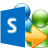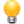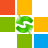# Find a Window by the Win32 Api

## This demonstrates how to get a window handle by the Win32 API.

◀ Previous sample Next sample ▶SAM Determine the "identity" of your emails. Set with SAM the sender and the folder folder for sent items with the help of rules.

The sample finds the window of a parent window by its caption and returns its handle. If you want to find a window of the top level, first call the GetDesktopWindow function and pass the result to the FindChildWindowText function. But you can also pass the handle of any other window to find one of its childs. Lower or upper cases don't matter; and you can search for exact matches or just a part of it by using a trailing asterisk (e.g. 'abc*').

Add a new module via Insert / Module, and paste the code there.How to add macros to Outlook
```Private Declare Function GetWindow Lib "user32" _
(ByVal hwnd As Long, ByVal wCmd As Long) As Long
Private Declare Function GetWindowTextA Lib "user32" _
(ByVal hwnd As Long, ByVal lpString As String, ByVal cch As Long) As Long
Private Declare Function GetDesktopWindowA Lib "user32" _
Alias "GetDesktopWindow" () As Long

Const GW_HWNDNEXT = 2
Const GW_CHILD = 5

Public Function GetDesktopWindow() As Long
GetDesktopWindow = GetDesktopWindowA
End Function

Public Function FindChildWindowText(ByVal lHwnd As Long, _
sFind As String _
) As Long
Dim lRes As Long
Dim sFindLC As String

lRes = GetWindow(lHwnd, GW_CHILD)
If lRes Then
sFindLC = LCase\$(sFind)
Select Case InStr(sFindLC, "*")
Case Is > 0
Do
If LCase\$(GetWindowText(lRes)) Like sFindLC Then
FindChildWindowText = lRes
Exit Function
End If
lRes = GetWindow(lRes, GW_HWNDNEXT)
Loop While lRes <> 0

Case Else
Do
If LCase\$(GetWindowText(lRes)) = sFindLC Then
FindChildWindowText = lRes
Exit Function
End If
lRes = GetWindow(lRes, GW_HWNDNEXT)
Loop While lRes <> 0
End Select
End If
End Function

Private Function GetWindowText(ByVal lHwnd As Long) As String
Const STR_SIZE As Long = 256
Dim sBuffer As String * STR_SIZE
Dim lSize As Long

sBuffer = String\$(STR_SIZE, vbNullChar)
lSize = GetWindowTextA(lHwnd, sBuffer, STR_SIZE)
If lSize > 0 Then
GetWindowText = left\$(sBuffer, lSize)
End If
End Function
```Category-Manager With Category-Manager you can group your Outlook categories, share them with other users, filter a folder by category, automatically categorize new emails, and more. You can use the Addin even for IMAP.